Algebra 2 6-4 Guided Practice: Rational Exponents
starstarstarstarstarstarstarstarstarstar
by Matthew Richardson
| 25 Questions1
2
1
10
Solve It! On the canvas, show how to cut the three linked 1-squares into congruent pieces, each with size ¾.
2
10
Solve It! Reasoning: Explain why you cannot cut them into congruent pieces, each with size 4/5.
You cannot cut the three 1-squares into congruent 4/5-pieces because 3 ÷ 4/5 is not a whole number.
You cannot cut the three 1-squares into congruent 4/5-pieces because 3 × 4/5 is not a whole number.
You cannot cut the three 1-squares into congruent 4/5-pieces because 3 is an odd number.3
4
5
3
10
Take Note: Write the expression below in radical form.

4
10
Take Note: Write the expression below in exponent form.

5
10
Take Note: Define principal root.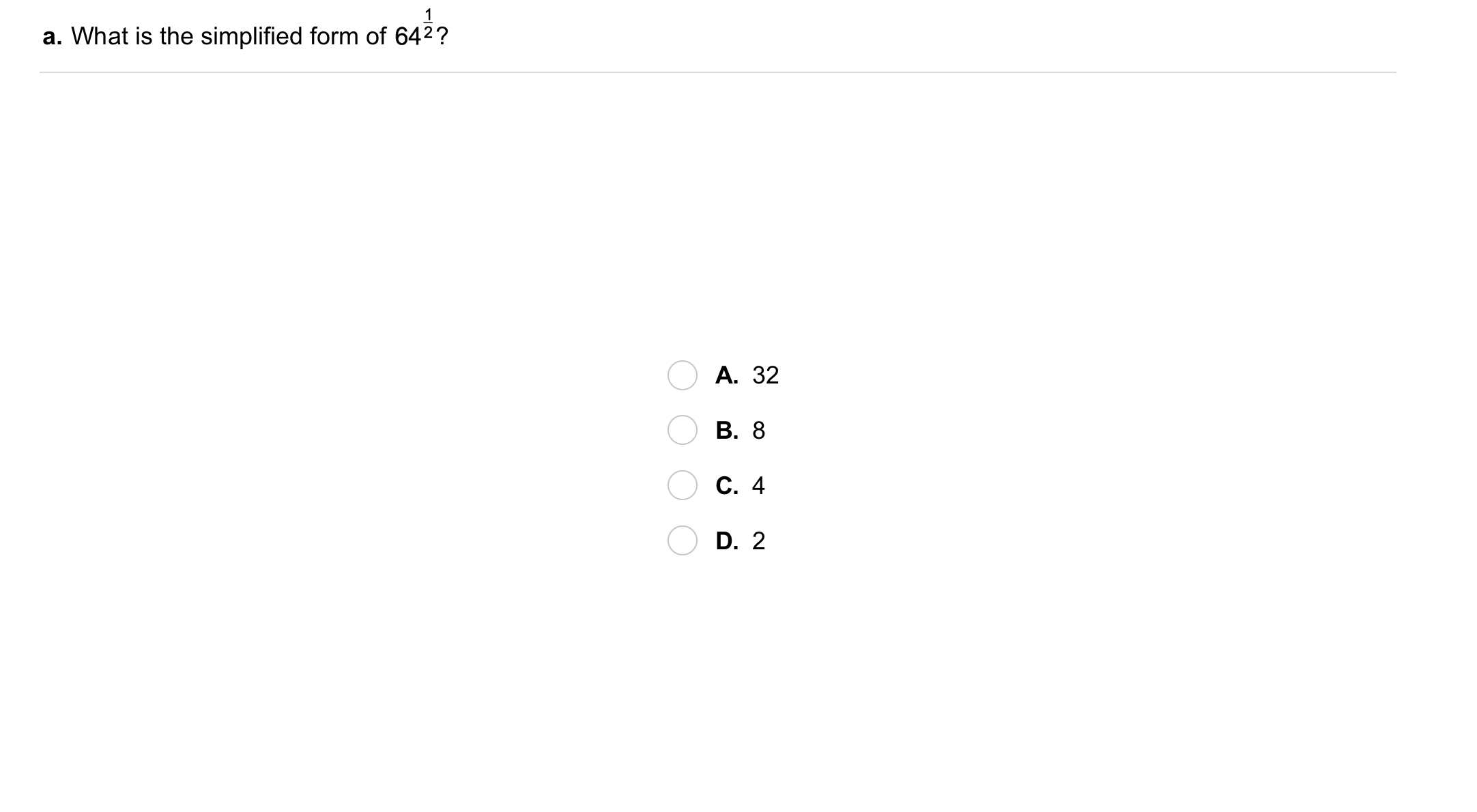6
6
10
Problem 1 Got It?
A
B
C
D7
7
10
Problem 1 Got It?
A
B
C
D8
8
10
Problem 1 Got It?
A
B
C
D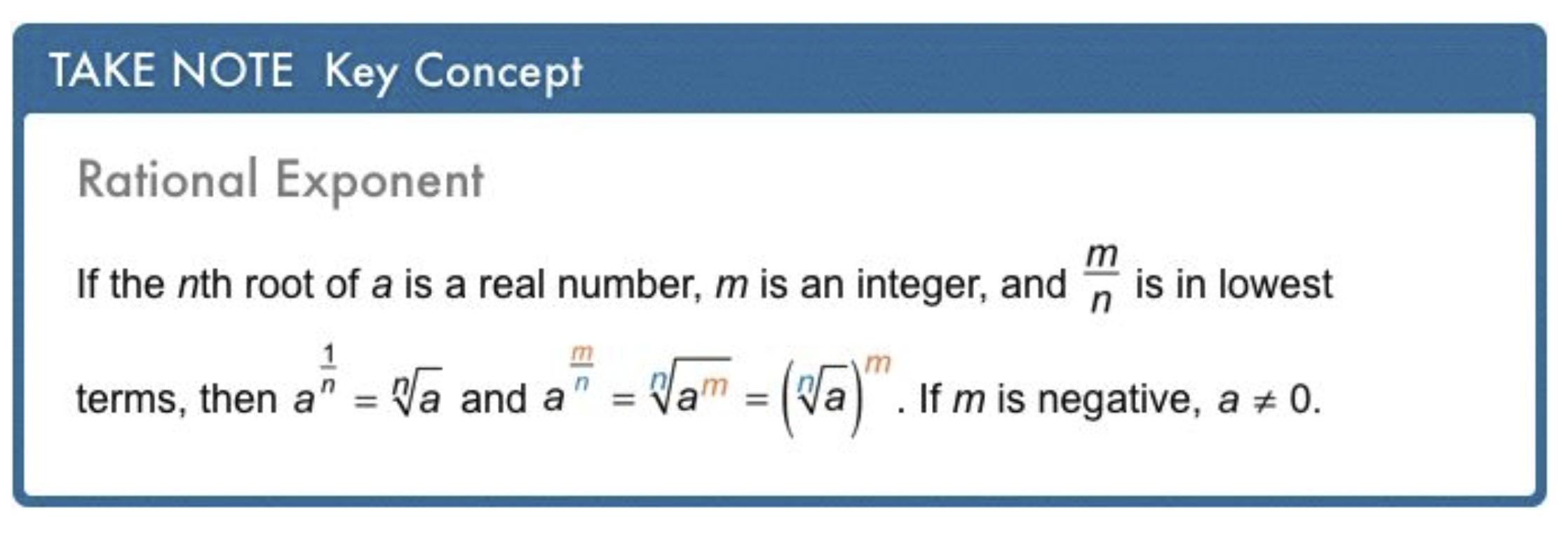9
10
Problem 2 Got It? What is the expression in radical form?

10
10
Problem 2 Got It? What is the expression in radical form?

11
10
Problem 2 Got It? What is the expression in exponential form?

12
10
Problem 2 Got It? What is the expression in exponential form?

13
10
Problem 2 Got It? Reasoning: Refer to the definition of rational exponent. Explain the need for the restriction that a ≠ 0 if m is negative. (In other words, if m is negative, why can't a be zero?)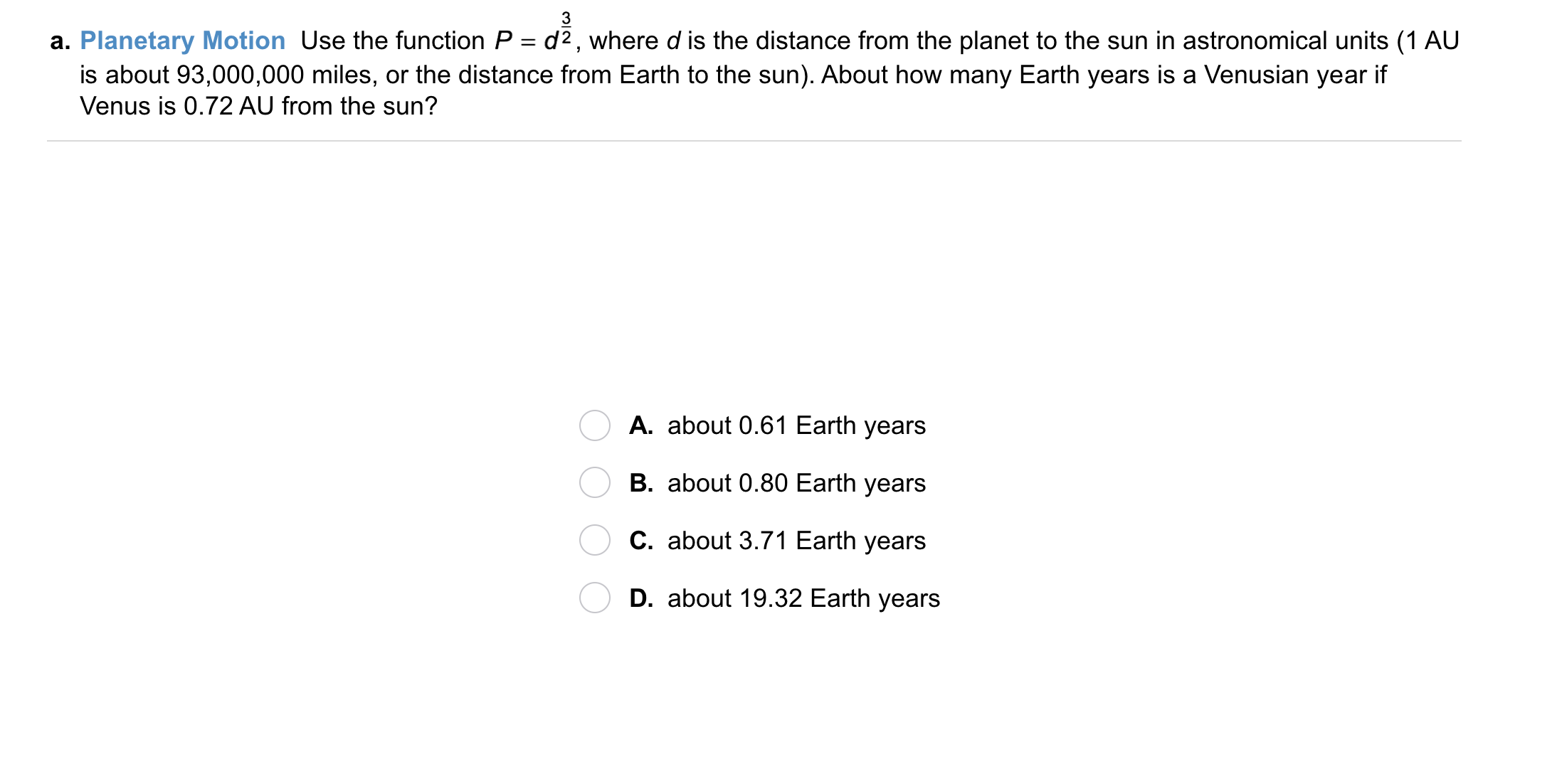14
14
10
Problem 3 Got It? Planetary Motion: Use the function
where d is the distance from the planet to the sun in astronomical units (1 AU is about 93,000,000 miles, or the distance from Earth to the sun). About how many Earth years is a Venusian year if Venus is 0.72 AU from the sun?
A
B
C
D15
15
10
Problem 3 Got It? Planetary Motion: Use the function
where d is the distance from the planet to the sun in astronomical units (1 AU is about 93,000,000 miles, or the distance from Earth to the sun). About how many Earth years is a Jovian year if Jupiter is 5.46 AU from the sun?
A
B
C
D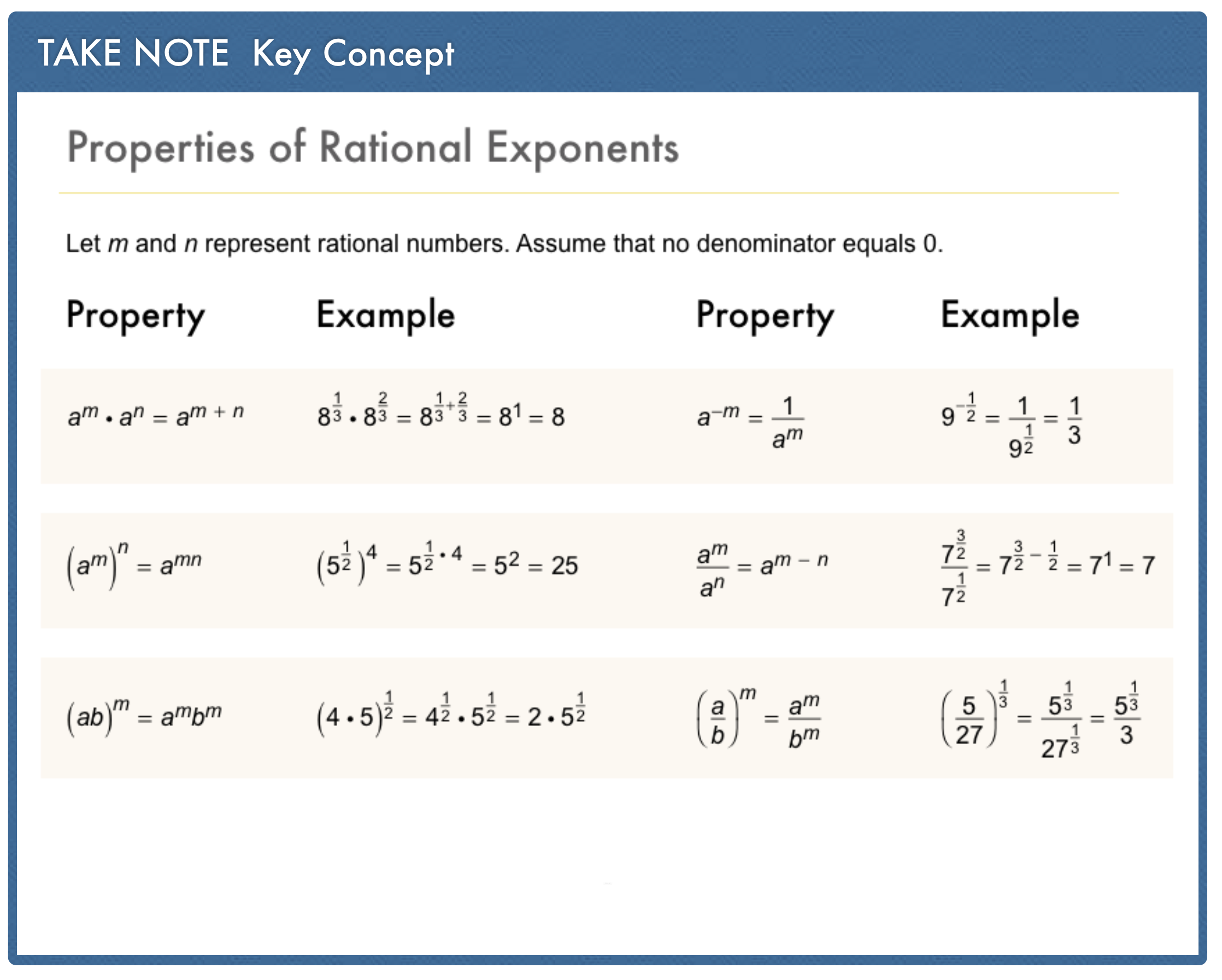a
Take Note: Take a moment to add the properties of rational exponents to your notes.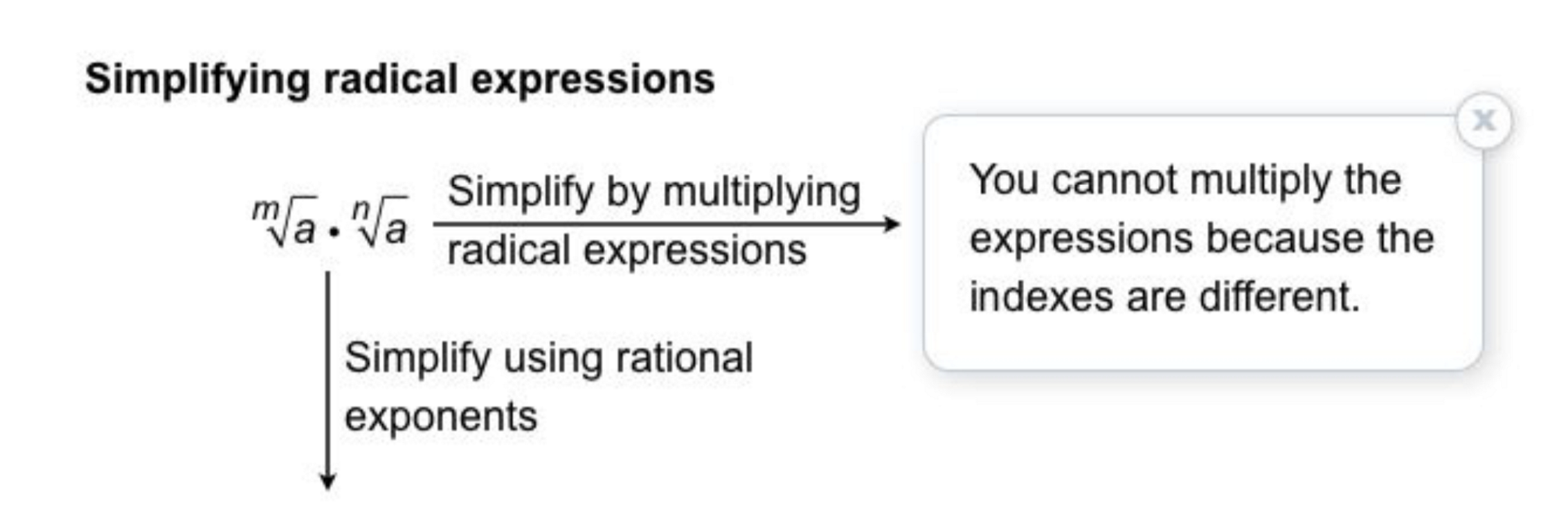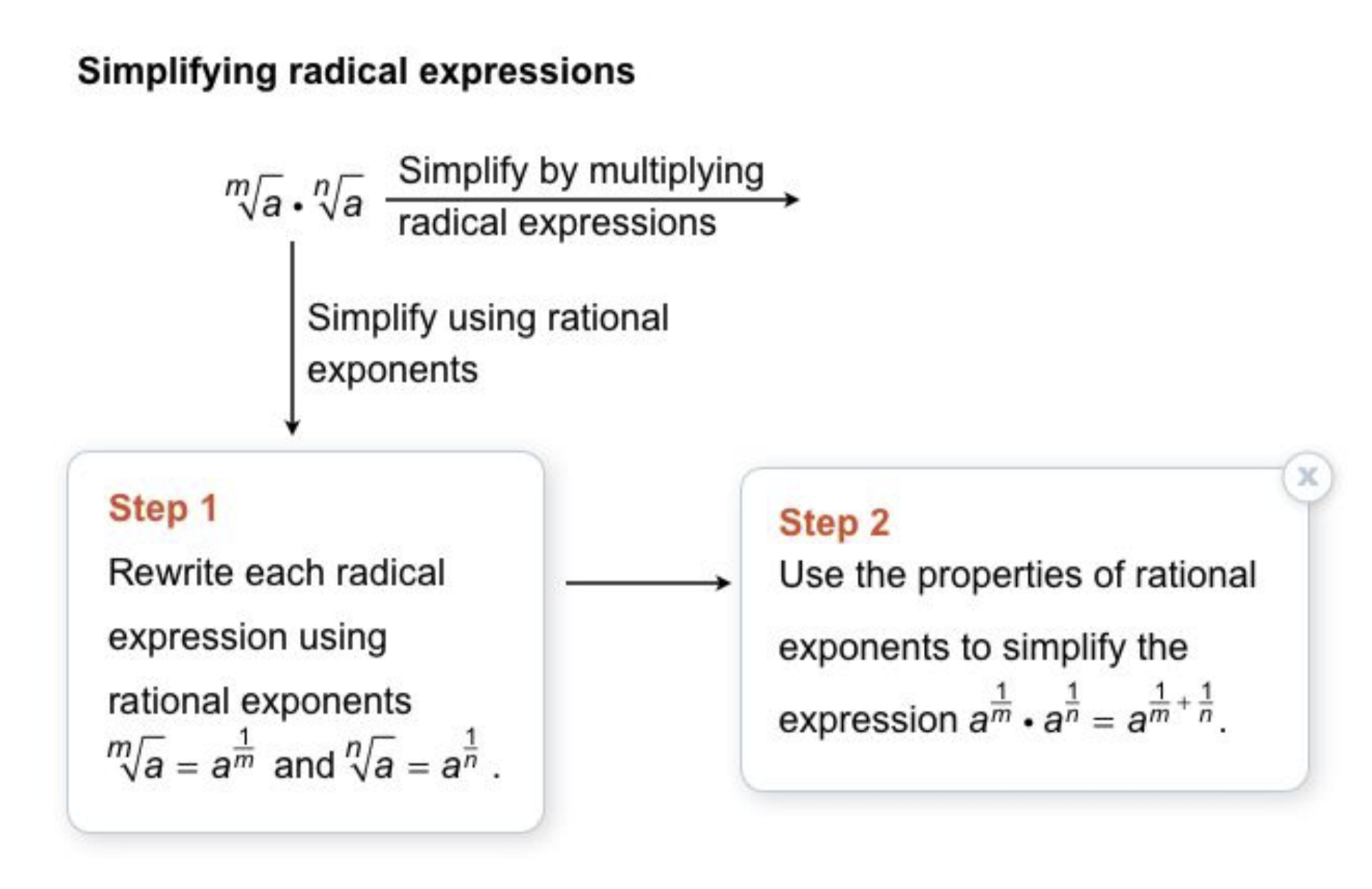16
16
10
Take Note: Describe the process of simplifying radical expressions.
◆ How do you know if you can simplify the expression in the first place?
◆ What are the 2 steps for simplifying the expression?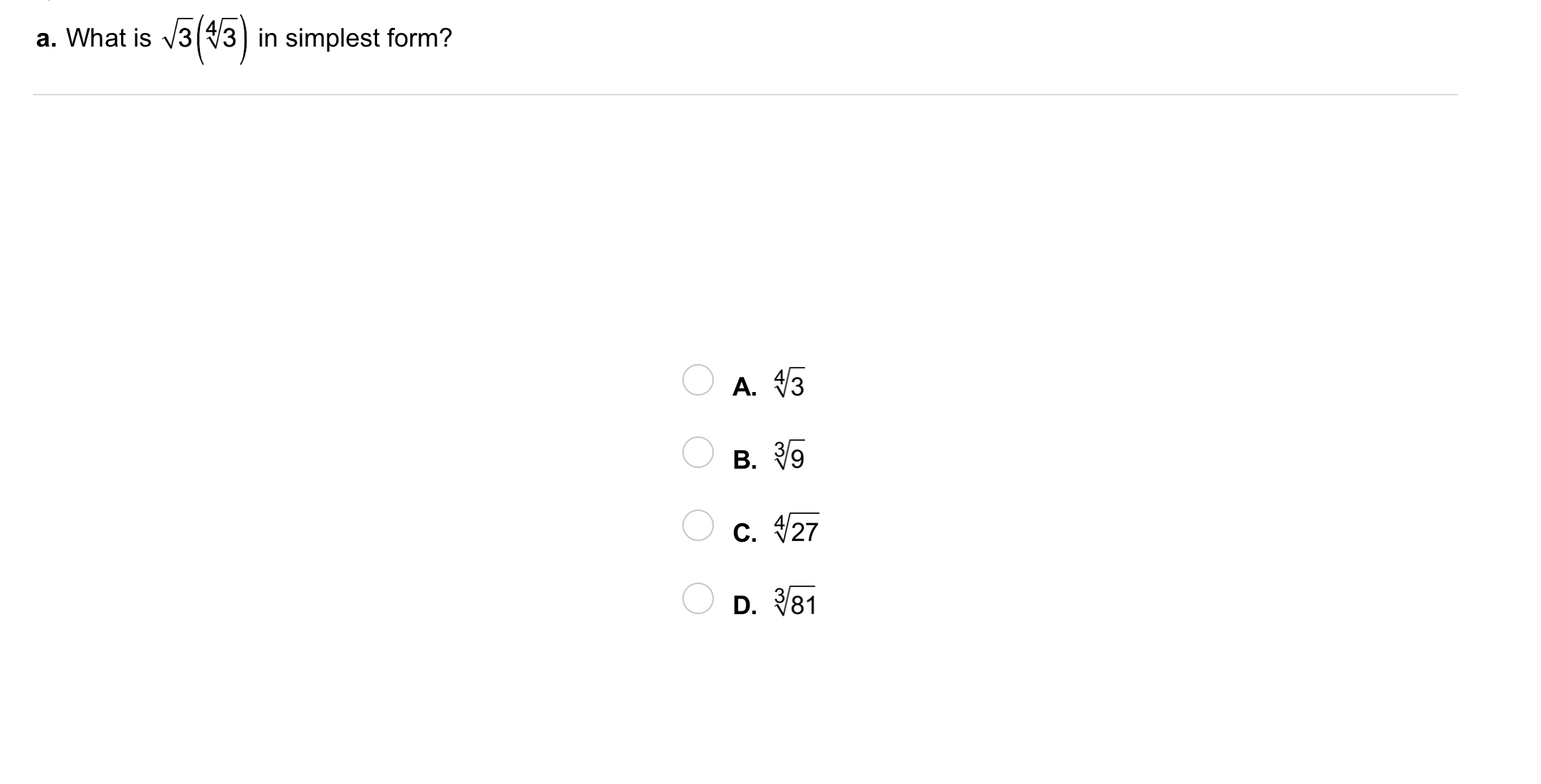17
17
10
Problem 4 Got It?
A
B
C
D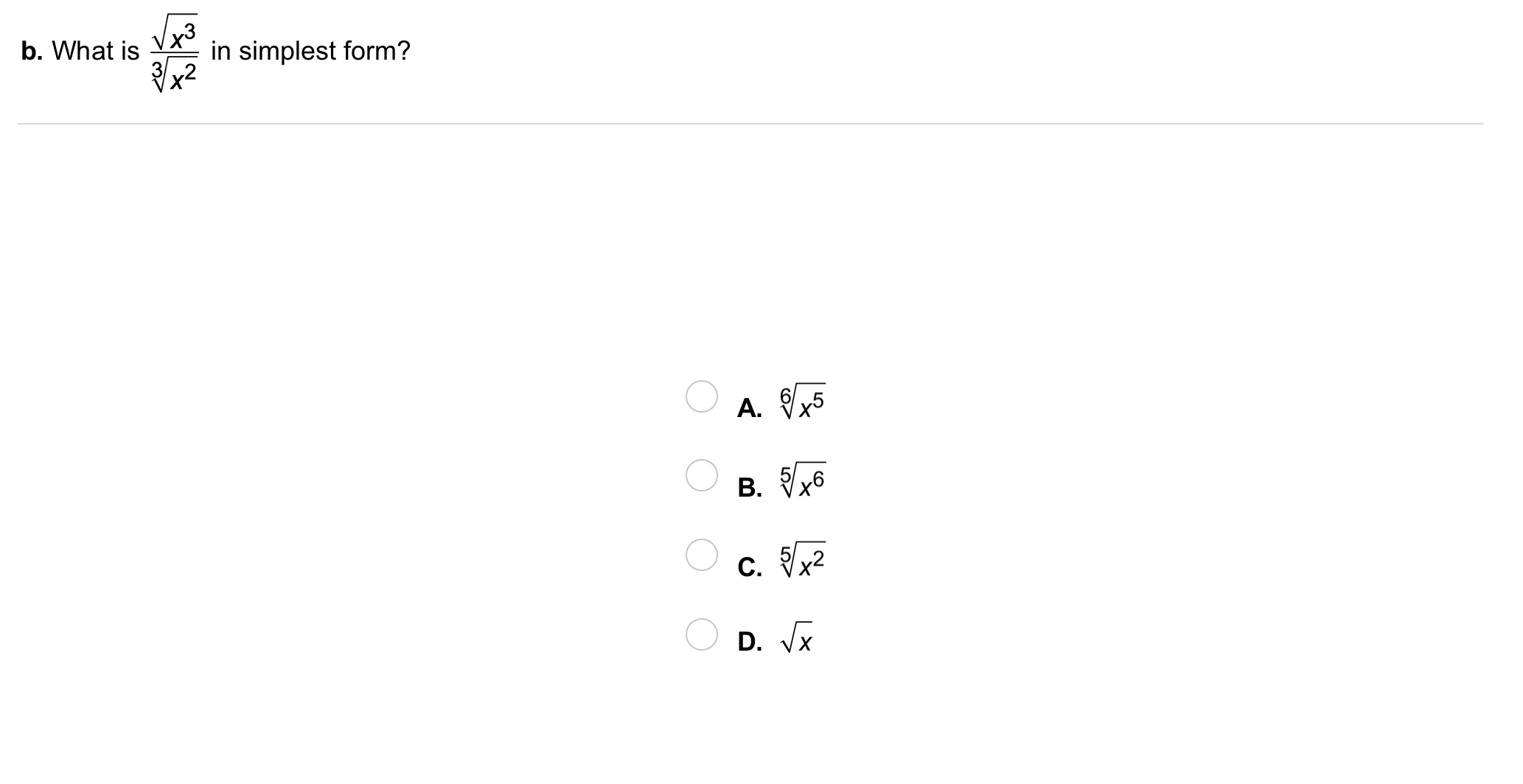18
18
10
Problem 4 Got It?
A
B
C
D19
19
10
Problem 4 Got It?
A
B
C
D20
20
10
Problem 5 Got It?
A
B
C
D21
21
10
Problem 5 Got It?
A
B
C
D22
22
10
Problem 5 Got It?
A
B
C
D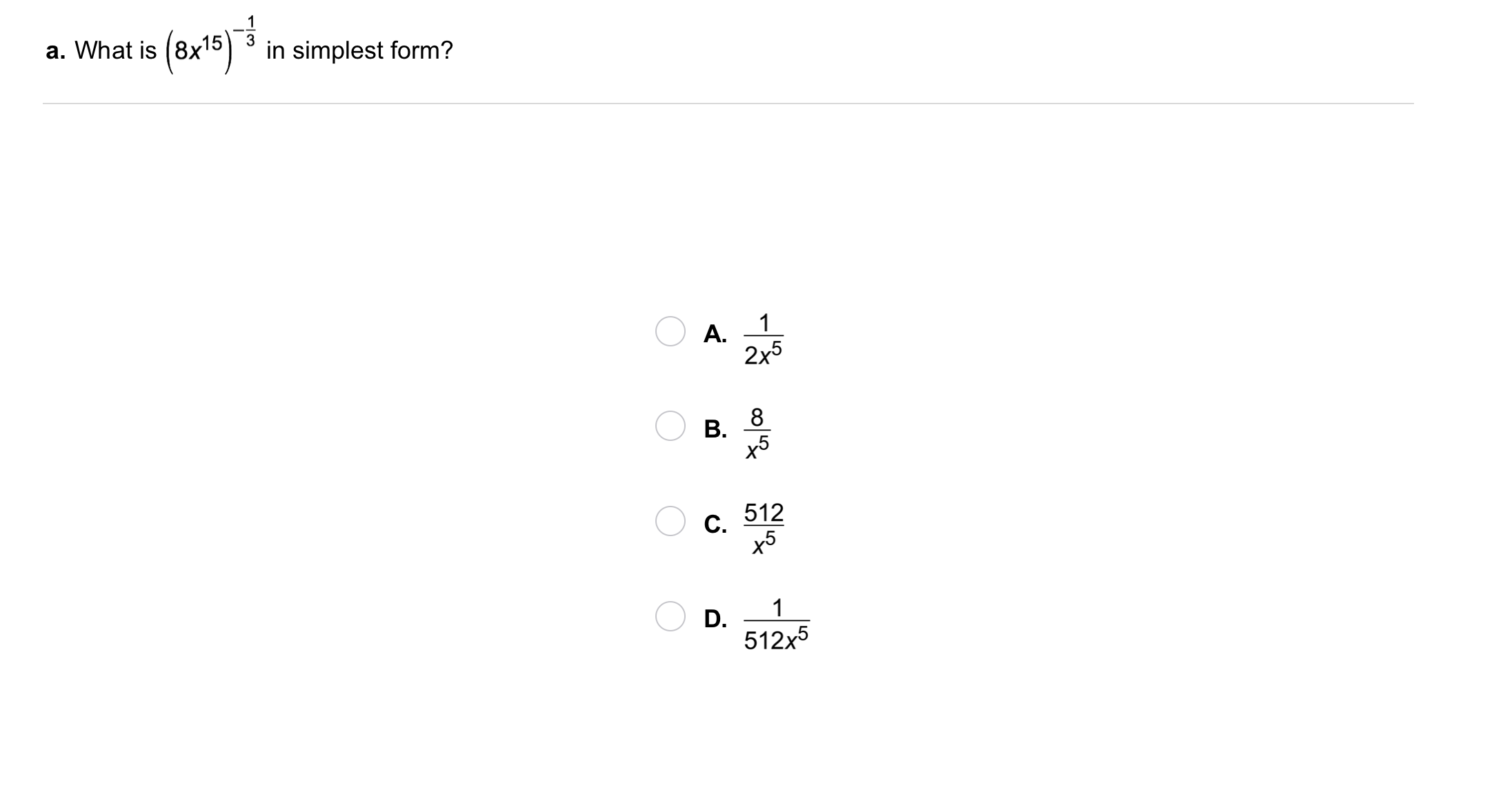23
23
10
Problem 6 Got It?
A
B
C
D24
24
10
Problem 6 Got It?
A
B
C
D25
25
10
Take Note: Summarize the content of this lesson.